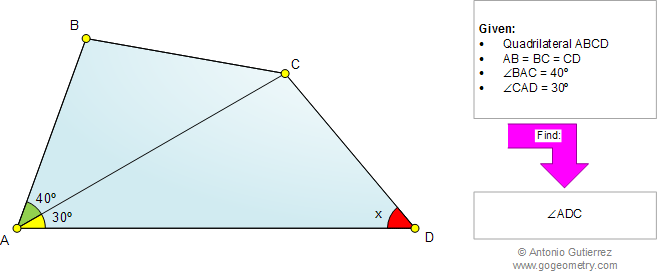v Math Education Geometry Problem 819: Quadrilateral, Triangle, Angles, 30 degrees. Level: High School, Honors Geometry, College, Mathematics Education. Distance learning.Online Geometry Problem 819: Quadrilateral, Triangle, Angles, 30 degrees. Level: High School, SAT Prep, College, Mathematics Education

 The figure below shows a quadrilateral ABCD with AB = BC = CD, angle BAC = 40 degrees, and angle CAD = 30 degrees. Find the measure of angle ADC. Try to use elementary geometry (Euclid's Elements).Home | Search | Geometry | Problems | All Problems | Open Problems | Visual Index | 10 Problems | 811-820 | Triangles | Quadrilaterals | Angles | 30 degrees | Email | Solution / comment | By Antonio Gutierrez